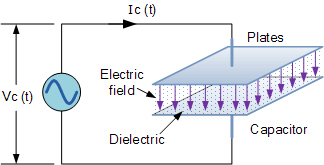## Capacitive Reactance Calculator

 I want to calculate --Select-- Frequency Capacitance Capacitive Reactance Frequency Hz Capacitance F Capacitive Reactance ohm

### Capacitive Reactance Formula

Xc = 1/(2πfC)

To Capacitance: C = 1/(2πXcC)

To Frequency: f = 1/(2πXcC)

Where, Xc = Capacitive Reactance in Ohms, (Ω), π (pi) = 3.1415 (decimal) or 22÷7 (fraction), ƒ = Frequency in Hertz, (Hz), C = Capacitance in Farads, (F)Thinkcalculator.com provides you helpful and handy calculator resources.